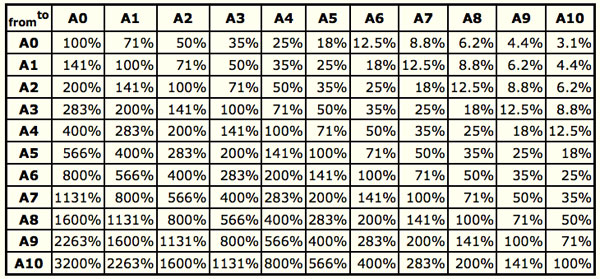# ISO Paper Sizes and Resizing A0 to A3I recently needed to reduce an A0 size file down to A3 and was confronted by loads of pages showing formulas for working this out.

But I wanted an easier way. Thankfully Markus Kuhn‘s website has a quick reference chart with ready-made resizing percentages.

The A series of paper sizes is based on these simple principles:

• The height divided by the width of all formats is the square root of two (1.4142).
• Format A0 has an area of one square meter.
• Format A1 is A0 cut into two equal pieces. In other words, the height of A1 is the width of A0 and the width of A1 is half the height of A0.
• All smaller A series formats are defined in the same way. If you cut format An parallel to its shorter side into two equal pieces of paper, these will have format A(n+1).
• The standardized height and width of the paper formats is a rounded number of millimeters.

For applications where the ISO A series does not provide an adequate format, the B series has been introduced to cover a wider range of paper sizes. The C series of formats has been defined for envelopes.